Percentages and indices. Secondary Education: 12 - 14 years.

Percentages.

Last July a department store reduced all their June prices on every item in the young person's clothes department by 15%. In June a pair of trousers cost 14.40. How much is this price reduced by? How much do the trousers cost in July?

Percentages are a special type of proportion or ratio. A 15% discount means that for every 100 of the price of an item the shop reduces it by 15. The total reduction is proportional to the original price. Therefore, to solve the problem we have to apply the following rule of three: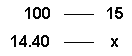Therefore, the reduction is x =  2.16. The final reduced price is 14.40 - 2.16 =  12.24

Percentages are probably most commonly used in everyday life to work out the direct proportion or ratio of something.

When solving problems with percentages the ratio is a quotient whose denominator is always 100. So in our example the ratio is 15/100 = 0.15. We can solve the problem by multiplying the original price by the ratio. In other words, the discount is 14.40 * 0.15 =  2.16

1. Use the following window to work out the reduction and sale price of these items in the same department: a shirt at 9, a jacket at 34.80, another pair of trousers at 19.20 and a polo shirt at 13.20. Write your results and draw a table like the one below into your notebook.

 Change the original price with the little arrows or type in the new value directly into the value box and press the ENTER key. You can solve other problems with different reductions by altering the percentage value.

 July sales Item Original price Reduction Sale price trousers 14.40 2.16 12.24 shirt 9.00 jacket 34.80 trousers 19.20 polo shirt 13.20

2. Just before the items were priced up with the new prices the department manager decided to increase the reduction to 20% instead of 15%. Work out the new reductions and sale prices for each of the items and write your results in the table below. Use the window above to check your results by changing the percentage value.

 July sales Item Original price Reduction Sale price trousers 14.40 shirt 9.00 jacket 34.80 trousers 19.20 polo shirt 13.20

3. At the end of July the department store decided to reduce their July prices by a further 20%. Work out how much each of the items should costs in August.

 August sales Item Original price July reduction Price in July August reduction Final sale price trousers 14.40 shirt 9.00 jacket 34.80 trousers 19.20 polo shirt 13.20

4. If we had reduced the prices in June by 40% would we have got the same sale prices in August as reducing the prices by 20% twice, as we did? Work it out and explain what you discover to your classmates.

More problems with percentages.

5. A school football team played 40 games in the school championship. They won 25, drew 10 and lost 5 games. What percentage of games did they win, draw and lose?

This problem is similar to previous examples. We have to apply the rule of three as follows: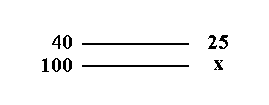So x= 25*100/40 = 62.5%

We can work out the percentage of games they drew and lost in the same way.

Check your results in the following window.

 Base is the number of games played. Value is the number of games won (or drawn or lost in each case).

6. There are 28 students in my class. The number of students that have passed their exams are as follows:

Work out what percentages of students have passed each subject. Use the window above to check your results.

 Maths 20 English 17 Natural sciences 19 Social sciences 24 Physical education 27

A more difficult problem.

7. The price of a jacket in a shop has been reduced by 20% and now costs  28.80. What was the original price before the reduction?

We can call the original price x. After the 20% reduction the jacket costs 80% of the original price. In other words 80% of x is equal to 28.80.

Work out the solution in your notebook and check your answer in the following window.

 You have to put the values for the sale price and the reduction into the window.

Value Added Tax (VAT).

Whenever you buy something the supplier adds what we call Value Added Tax (or VAT) to the price of the item which they then pay to the tax office. This tax is a certain percentage of the price of the item. The percentage changes depending on what the item is you are buying. For example, if you buy a television set or a computer game VAT is 16% of the price but if you buy a book it is only 7%.

We are going to look at an example: if you buy a computer that costs 720 in a catalogue you have to work out 16% of this price to calculate how much the VAT is. Therefore, the amount paid in VAT is

720*16/100 = 115.20

so when we add this to the basic price we get 835.20 which is the total price.

The percentage is added to the original price to find out the total amount we have to pay.

8. Work out the VAT and total prices of the following items given the original prices and the amount of VAT we have to add. Write your results in the following table:

 Item Price before VAT VAT rate Amount of VAT Total price A light bulb 0.75 16% A book 13.80 7% Electricity bill 18.36 16% A loaf of bread 0.31 2% A pen 7.20 16%

Check your results in the following window.

 You have to put in the prices before VAT and the VAT rates given into the window.

9. An item, which has had 16% VAT added to the original price, is sold for 63.00. What is the original price before VAT was added? Check your result in the window above.

 Simple interest. Different banks pay their customers a certain amount of money each year which is proportional to the amount they have in their bank accounts or savings accounts. This quantity of money is called interest and is worked out at a certain percentage. Let's look at an example: Isabel has 3,000.00 saved in a savings account that offers her an annual interest rate of 2.5%. How much interest will she earn on the capital after a year? What about after 3 years?The interest rate is 2.5% which means that for each 100 that she has saved she earns 2.50 a year. So 2.5% of 3,000 is: 3,000 * 2.5 / 100 =  75.00  After three years she will earn 3 times this interest, which is: 3,000 * 2.5 / 100 * 3 =  225  In general, if c is the capital paid into the account, r the interest rate (also referred to as the return) and t the time that interest i is earned on the money we get the following formula: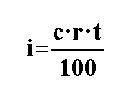10. Work out the interest made on 4,200 at an interest rate of 6.25% over a period of 5 years. 11. Henry pays some money into a bank account that gives him an annual interest rate of 3.75%. After two years he has 222 in the account. How much money did he have in his account to start with? Check your results in the following window:

 The retail price index (RPI). The RPI is a measurement that shows the changes (increase or sometimes decrease) in the cost of basic goods and services. This index is calculated as a percentage. So, when newspapers on the 10th of the month publish that the RPI has increased by two tenths (0.2%) this means that prices have risen by this percentage since last month. This doesn't mean that all goods (food, petrol, electricity, housing) go up by this amount. The RPI is an average measurement of the increase of all prices since the previous month.The RPI is a very important measurement as it is used as the main indicator of inflation and as a point of reference to increase salaries every year. 12. The government predicts that by the end of 1998 the RPI in 1999 will increase by 1.8%. Therefore, they decided to increase all civil servants' salaries by this amount. Louise, Peter and Albert's  parents are all civil servants and in 1998 earned a monthly salary of 1000, 1200 and 1350 respectively. How much more will they each earn and what will their new monthly salary be in 1999? If the government had predicted that the RPI was going to increase by 2.1% instead, what would their monthly salaries have been increased to in 1999? Check your results in the following window: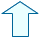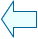Fernando Arias Fernández-PérezSpanish Ministry of Education. Year 2001